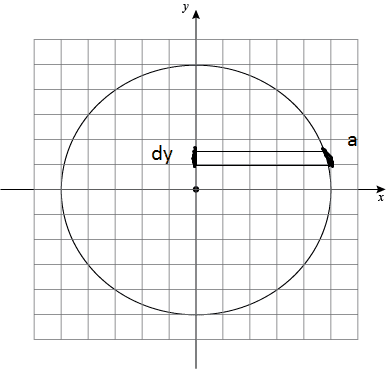# Where is wrong in this proof for rotational inertia ?

## Homework Statement

Prove the formula for inertia of a ring (2D circle) about its central axis.

## Homework Equations

$$I = MR^2$$
Where:
M: total mass of the ring

## The Attempt at a Solution

- So I need to prove the formula above.

- First, I divide the ring into 4 section so I only need to calculate the rotational inertia of the first quadrant (which has only positive coordinates), then multiply by 4.

- Then, I divide the quadrant into infinite arc length, each is determined by an interval on Oy axis. Each interval is determined by its position y and its height dy. So I integrate along the Oy axis.
+ Since dy is very small, dy ≈ arc length.
+ The differential mass dm then equals: $$dm = \frac{dy}{2 \pi R}M$$
* Explanation: $$\frac{dy}{2 \pi R}$$ is the portion of the ring that belongs to this arc length.​
+ This mass has distance R to the central axis.
→ The rotational inertia for this mass is: $$dI = \frac{dy}{2 \pi R}MR^2$$

→ The rotational inertia for ring is:
$$I = 4 \int_{0}^{R}\frac{dy}{2 \pi R}MR^2$$

- Solving for this, I get $$I = \frac{2MR^2}{\pi}$$

4. Conclusion:
- Could someone tell me where I went wrong with my calculation ? Please show me the mistake and correction but not an entire different solution.
This is the first time I ask a question on physicsforum, so please forgive me if there're any mistakes.

Last edited:

cnh1995
Homework Helper
Gold Member
Ho Duke! Welcome to PF!
dm=dy2πRM
This is unnecessary.

You have assumed the ring to be made up of infinite small masses 'dm'. All these masses are equidistant from the center. What is the moment of inertia of individual dm about the central axis? This would be much simpler.
Please show me the mistake and correction but not an entire different solution.
Ok. If you want to use your method, your integration limits are wrong. You are moving "along" the ring, not from 0 to R. So what should be the upper limit of y? Is this hint enough?

Ho Duke! Welcome to PF!
Ok. If you want to use your method, your integration limits are wrong. You are moving "along" the ring, not from 0 to R. So what should be the upper limit of y? Is this hint enough?

I think I have found the mistake. My intention for integrating from 0 to R was that dy ~= arc length from y to y+dy (moving vertically in y+ direction), I guess that approximation is wrong here :D

The upper limit should be R*pi/2

Last edited:
PeroK
Homework Helper
Gold Member
2020 Award
I think I have found the mistake. My intention for integrating from 0 to R was that dy ~= arc length from y to y+dy (moving in y+ direction), I guess that approximation is wrong here :D

The upper limit should be R*pi/2

This is why polar coordinates were invented!

This is why polar coordinates were invented!

I haven't really learned polar coordinate yet, only its definition and simple calculation. I did try integration using dθ and Rcos(θ) though, it was:
$$I = 4\int_{0}^{\frac{\pi }{2}} \frac{d\theta }{2 \pi}MR^2$$
and it gave the correct result. I just had no idea why the other formula didn't work.

Is the approximation dy ≅ arc length from y to y + dy ever used ? Or is it just wrong ? Thank you.

PeroK
Homework Helper
Gold Member
2020 Award
Is the approximation dy ≅ arc length from y to y + dy ever used ? Or is it just wrong ? Thank you.

It's not an approximation. When ##y## is close to 1, then ##\Delta y \approx 0##.

It's not an approximation. When ##y## is close to 1, then ##\Delta y \approx 0##.

I'll add a picture because I don't have enough vocabulary yet.For the dy ≅ arc length approximation, I meant: dy ≅ a when dy is very small.
Is this approximation valid ?

PeroK
Homework Helper
Gold Member
2020 Award
I'll add a picture because I don't have enough vocabulary yet.
View attachment 112532

For the dy ≅ arc length approximation, I meant: dy ≅ a when dy is very small.
Is this approximation valid ?
No.

No.

Okay that's where things went wrong. Thanks a lot !!